# pylops.signalprocessing.Convolve2D#

class pylops.signalprocessing.Convolve2D(dims, h, offset=(0, 0), axes=(-2, -1), method='fft', dtype='float64', name='C')[source]#

2D convolution operator.

Apply two-dimensional convolution with a compact filter to model (and data) along a pair of axes of a two or three-dimensional array.

Parameters
dims

Number of samples for each dimension

hnumpy.ndarray

2d compact filter to be convolved to input signal

offsettuple, optional

Indices of the center of the compact filter

axesint, optional

New in version 2.0.0.

Axes along which convolution is applied

methodstr, optional

Method used to calculate the convolution (direct or fft).

dtypestr, optional

Type of elements in input array.

namestr, optional

New in version 2.0.0.

Name of operator (to be used by pylops.utils.describe.describe)

Notes

The Convolve2D operator applies two-dimensional convolution between the input signal $$d(t,x)$$ and a compact filter kernel $$h(t,x)$$ in forward model:

$y(t,x) = \iint\limits_{-\infty}^{\infty} h(t-\tau,x-\chi) d(\tau,\chi) \,\mathrm{d}\tau \,\mathrm{d}\chi$

This operation can be discretized as follows

$y[i,n] = \sum_{j=-\infty}^{\infty} \sum_{m=-\infty}^{\infty} h[i-j,n-m] d[j,m]$

as well as performed in the frequency domain.

$Y(f, k_x) = \mathscr{F} (h(t,x)) * \mathscr{F} (d(t,x))$

Convolve2D operator uses scipy.signal.convolve2d that automatically chooses the best domain for the operation to be carried out.

As the adjoint of convolution is correlation, Convolve2D operator applies correlation in the adjoint mode.

In time domain:

$y(t,x) = \iint\limits_{-\infty}^{\infty} h(t+\tau,x+\chi) d(\tau,\chi) \,\mathrm{d}\tau \,\mathrm{d}\chi$

or in frequency domain:

$y(t, x) = \mathscr{F}^{-1} (H(f, k_x)^* * X(f, k_x))$

Methods

 __init__(dims, h[, offset, axes, method, ...]) adjoint() apply_columns(cols) Apply subset of columns of operator cond([uselobpcg]) Condition number of linear operator. conj() Complex conjugate operator div(y[, niter, densesolver]) Solve the linear problem $$\mathbf{y}=\mathbf{A}\mathbf{x}$$. dot(x) Matrix-matrix or matrix-vector multiplication. eigs([neigs, symmetric, niter, uselobpcg]) Most significant eigenvalues of linear operator. matmat(X) Matrix-matrix multiplication. matvec(x) Matrix-vector multiplication. reset_count() Reset counters rmatmat(X) Matrix-matrix multiplication. rmatvec(x) Adjoint matrix-vector multiplication. todense([backend]) Return dense matrix. toimag([forw, adj]) Imag operator toreal([forw, adj]) Real operator tosparse() Return sparse matrix. trace([neval, method, backend]) Trace of linear operator. transpose()

## Examples using pylops.signalprocessing.Convolve2D#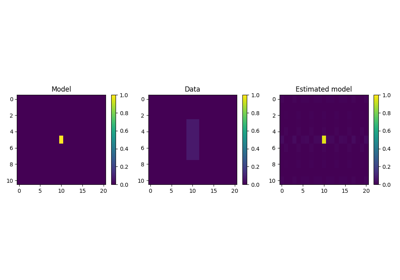2D Smoothing

2D Smoothing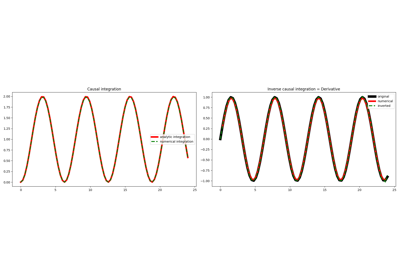Causal Integration

Causal Integration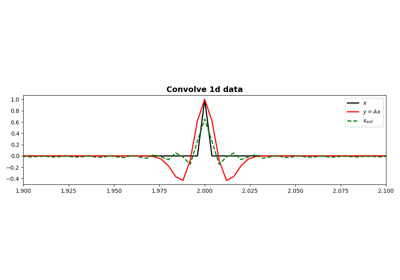Convolution

Convolution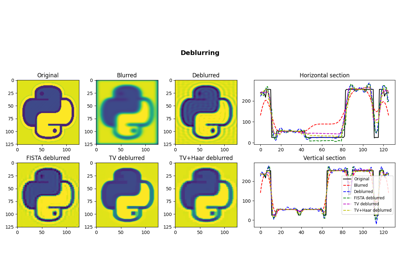05. Image deblurring

05. Image deblurring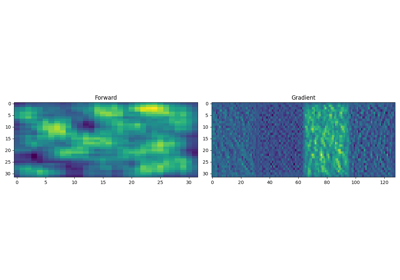19. Automatic Differentiation

19. Automatic Differentiation